Search IntMath
Close

450+ Math Lessons written by Math Professors and Teachers

5 Million+ Students Helped Each Year

1200+ Articles Written by Math Educators and Enthusiasts

Simplifying and Teaching Math for Over 23 Years

# What is 0^0 equal to?

By Murray Bourne, 22 Jan 2009

In the introduction to Laws of Integral Exponents, I mention the debate about the value of 00. Is it zero or is it one?

Why is it a problem? Look at the following 2 patterns:

Multiply 0 as many times as you like, you get 0.

0^3 = 0
0^2 = 0
0^1 = 0
0^0 = 0

But then again, any number raised to the power 0 is 1:

3^0 = 1
2^0 = 1
1^0 = 1
0^0 = 1

That's why there is dispute about the value of 0^0.

On the page linked to before, I wrote:

It is most commonly regarded as having value 1

An interesting conundrum. Sometimes our wonderful, (normally) consistent system of math breaks down.

### 42 Comments on “What is 0^0 equal to?”

1. Susan says:

Ah....

x^0=1 because of the rule x^s divided by x^t = x^(s-t)

If s is larger than t, we have more x in the numerator, so with canceling all of the common x's we are left with (s-t) in the numerator.

For example: x^5 divided by x^3 we have (x*x*x*x*x) divided by (x*x*x) so we can cancel three of the x's in the numerator and three in the denominator (because x divided by x = 1)
leaving two x's remaining, and therefore x^2

But if we have the same number of x's in numerator and denominator then we can cancel all of them - or divide all of them leaving 1*1*1*1... which is clearly 1.

It is not convention that makes this true. It is what follows from the basic laws of algebra.

x^0= 1 is not a convention, it is a consequence.

2. Murray says:

Thanks, Susan

But if x = 0, we cannot divide as you are suggesting, because dividing by 0 (or any power of 0) is undefined.

3. Susan says:

Exactly!
0^0 is not a conundrum it is an impossibility!
Because dividing by 0 doesn't make sense!

When I teach dividing by 0 as impossible, I say to my students -

Suppose there are 20 students in class and I brought in 40 donuts how many would each student get? They say easy - 2 because 40/20=2

So I say on a day like today, when I bring 0 donuts to divide up, how many do the 20 students get, and logically the answer is none because 0/20=0

Ah but if I show up for class and none of my students show up, then what do I do? I can't dive my 20 donuts up between 0 students! It's impossible. Plus - if none of them show up, they're in big trouble!

It's those little math stories that help what seems mystical and tricky just seem common sense!

4. Saagar says:

Nice one Susan, I like the way you interpret it. I am not Math teacher myself but such math questions always intrigue me. A quick question to both you and Zac. Are there any significantly known patterns in Prime numbers. I worked with a Prof for some time in college on that for a little time, but never had time to see it through..

5. Eman says:

I personally don't think that stories and common sense are a way to decide something in math. Complex numbers don't make sense or fit any story but they are valid nonetheless.
A different way to approach this problem is using L'Hopital's rule: lim x->0 of x^x = y
take the natural log of both sides:
lim x->0 x ln x = ln y
lim x->0 lnx/(x^-1) = lim x->0 (1/x)/(-x^-2) = lim x->0 -x = 0 = ln y
y = 1 = lim x->0 x^x

6. amir says:

hi Eman
the way u interpret the result is great
but i am a little confused about the step(2nd last)
lim x->0 lnx/(x^-1) = lim x->0 (1/x)/(-x^-2) = lim x->0 -x = 0 = ln y
can u kindly simply brief it again..............
thanks a lot.

7. Alan Cooper says:

Hi Zac,
Thanks for posting this intriguing conundrum. It is interesting to consider why most mathematicians use 0^0=1 as well as whether or not the possibility of alternative choices represents a breakdown of consistency in mathematics.
The argument that 0^3=0,0^2=0,0^1=0 (etc) imply 0^0=0 does, at first, seem just as convincing as the other one, but actually it is a bit "one-sided", since one could just as well say 0^(-3) is undefined (or "infinite" if you like to put it that way) and ditto for 0^(-2), 0^(-1), etc, so why shouldn't the same be true for 0^(-0) (which is of course the same as 0^0)?

On the other hand, x^0=1 is true not just for positive x but also negative ones (and in fact for all nonzero complex numbers). So to extend the definition by defining 0^0=1 has the advantage of creating a continuous extension of x^0 to all real (and complex) numbers.

So x^0=1 can be usefully extended in a continuous way to all numbers but 0^x=0 cannot. This is why mathematicians generally adopt the 0^0=1 definition. But of course it remains just a definition - as is each step in the process of extension from the more primitive cases of b^p where b and p are both positive integers and where the power is defined by repeated multiplication.

In fact, it often happens that a concept or operation defined in a restricted context has more than one reasonable extension to a broader context and so we can't use both without giving them different names. Often people are careless and use the same name for the extended operation and it is usually not a problem because everyone makes the same choice. But if different people make different choices and both fail to change the name then this does lead to an inconsistency. I guess whether or not this is a breakdown of the consistency of mathematics depends on whether by "mathematics" we mean the set of statements made by mathematicians or the fundamental reality that those statements are intended to describe. Perhaps we should cconsider the existence of alternative interpretations for 0^0 as an inconsistency of mathematicians than of mathematics.

cheers,
Alan

P.S. With regard to the wording of the "repeated multiplication" definition, wouldn't "multiply b by itself p times" give us b^(p+1)? (or perhaps b^(2^p) if you interpret it another way?).
Personally I prefer the clumsier-sounding (but clearer) "multiply together p copies of b".

8. alQpr » Blog Archive » What is 0^0 equal to? - squareCircleZ says:

[...] This post at squareCircleZ (a very nice enrichment and support website for students and teachers of mathematics) raises the conundrum of how to define 0^0 if all positive x give x^0=1 and 0^x=0. It is indeed a useful exercise for students to consider why most mathematicians use 0^0=1 as well as whether or not the possibility of alternative choices represents a breakdown of consistency in mathematics. [...]

9. Murray says:

Saagar: Here are some articles on the spacing of prime numbers:
http://mathforum.org/library/drmath/view/68490.html

10. Murray says:

Amir: This is what Eman was doing. I hope this is easier to read:

Let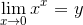Take log both sides: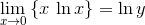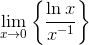Applying l'Hopital's rule, which says that the limit of the derivative of numerator and denominator will be the required limit: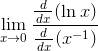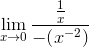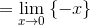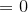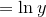So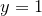Since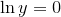and we can conclude: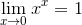giving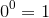11. Susan says:

Eman,
Saying L'Hopital's rule to one first leaning algebra is akin to saying,"Because I said so." Isn't that why so many think math doesn't make sense.

Indeed, it does.

12. Alan Cooper says:

With regard to Eman's comment: although x^x does approach 1as x->0+, there are other expressions of the form x^y which approach different limits as (x,y)->(0,0) with x positive.
[For example x^(-1/ln(x)) has a limit of 1/e, and x^(-1/sqrt(ln(x))) has a limit of zero.]
So to make the choice of 0^0=1 convincing I think we do need to consider negative x (which means we can't also continuously vary the exponent without introducing complex numbers).

13. Burton MacKenZie says:

a^0 = 1; for a !=0 only

0^0 is not defined.

References:

1. "Standard Mathematical Tables", 28th Edition, CRC Press, Inc, 1987, page 7.

2. "Technical Mathematics with Calculus", 4th Edition, Calter & Calter, John Wiley & Son, Inc, 2000, page 61.

3. "Algebra and Trigonometry", 4th Edition, Margaret Lial, Scott Foresman & Company, 1986, page 18.

14. Daniel says:

well i just yped 0^0 into my graphics calculator, and i got 1.

So that's the final word.

15. sohith says:

0^0 cannot be equal to 1 since according to laws of exponents, we derive a^0 by definition that a is not equal to 0.
a^m / a^m leads to a^m-m = a^0 =a^m /a^m = 1. But this is not possible if a is zero . Hence 0^0 cannot be equal to 1.

But if we want to apply that law to be equal to 1, then what we get is indefinite form of 0/0. Hence I think we cannot say the value of 0^0 =1 . WE cannot even say that 0^0 =0 since if x^x = x then we would get x=1 and x=0 but we could see that it would lead to x^x-1 = 1 which would make impossible if we substitute x=0. Hence as far as I know 0^0 is undefined.

16. Greg says:

I am not a deep thinker, so I suspect there will be big holes in my ideas here. Still, I foolishly press on...
I do believe I understand the prohibition on dividing by zero, in that dividing is the, um, inverse (or is it reverse?) operation of multiplication, and a times zero = b cannot be algebra'd into a = b divided by zero.
And I see the point about distributing doughnuts to zero students.
Still, one may think of division as: "How many times can I take a out of the number b?" Under this thought process, one might say: I can take zero out of any number an infinite number of times, so any number divided by zero equals infinity. (Infinity is not a number, though...) Likewise, I could say "I can hand out 20 doughnuts to zero students an infinite number of times, because after each round of handing out doughnuts, I still have 20 doughnuts left to hand out!"
Sheesh.

17. Eman says:

The alternating sum of binomial coefficients from the n-th row of Pascal's triangle is what you obtain by expanding (1-1)^n using the binomial theorem, i.e., 0^n. But the alternating sum of the entries of every row except the top row is 0, since 0^k=0 for all k greater than 1. But the top row of Pascal's triangle contains a single 1, so its alternating sum is 1, which supports the notion that (1-1)^0=0^0 if it were defined, should be 1.

1
1 1
1 2 1
1 3 3 1
etc. etc.
if you alternate the elements, they equal zero. Except for the top, which is 1.

18. ENG:USMAN says:

Thanks a lot. Plz give me knowledge about "numerical analysis". Thanks a lot.

19. Alan Cooper says:

Actually I agree with Burton MacKenZie that most mathematicians would say 0^0 is unefined, so the references to "most mathematicians" in my previous comments should (at most) have said "some" rather than "most".

20. Alan Cooper says:

Now the colleague whose siding with Burton persuaded me to retract my "most" is starting to change his tune!

After checking out some other references - such as listed at http://mathforum.org/dr.math/faq/faq.0.to.0.power.html - he may be entering the 0^0=1 camp - or at least admitting that it was bigger than he thought. (But of course the question of how many make which choice is more one of the sociology of mathematics than of mathematics itself.)

21. Burton MacKenZie says:

Alan, here's another good reference to get you thinking about 0^0:

http://mathworld.wolfram.com/Power.html

"0^0 (zero to the zeroth power) itself is undefined. The lack of a well-defined meaning for this quantity follows from the mutually contradictory facts that a^0 is always 1, so 0^0 should equal 1, but 0^0 is always 0 (for a>0), so 0^0 should equal 0. The choice of definition for 0^0 is usually defined to be indeterminate, although defining 0^0=1 allows some formulas to be expressed simply (Knuth 1992; Knuth 1997, p. 57)."

22. Murray says:

Burton

but 0^0 is always 0 (for a>0),

should be

but 0^a is always 0 (for a>0)

Also, this does not follow, since it said a>0

so 0^0 should equal 0

Actually, this is a pretty strange definition:

The choice of definition for 0^0 is usually defined to be indeterminate

Did Knuth actually write that?

23. mn says:

0^0 = 0/0
0^0 represents 0/0 in exponent form.

3^0 = 3/3
2^0 = 2/2
1^0 = 1/1
0^0 = 0/0
(-1)^0 = (-1)/(-1)

2*2*2*2*2 restated to make life easier 2^5
1/(2*2*2*2*2) restated to make life easier 2^(-5)

Exponent forms simply represent multiplication and division.

Depending on the usage of your denominator:
0/(0 groups) = no value
0/(0 items per group) = all values possible

24. dave says:

is dat tru when thy say ln(a)divided by ln(b) is not equals to ln(a/b)??????????

25. Murray says:

Hi Dave. Yes, it is true that log(a)/log(b) is not equal to log(a/b) (except for certain values of "a" and "b", like a = 1.

In fact, log(a/b) = log(a) - log(b). See Log Laws

26. Sarbajit Das says:

I think we should consider the value of 0^0 to be undefined.
I am giving examples to explain this case.

let, B not equal to 0 (!= 0).
B is a variable which can hold value of any integer at a time, except 0.

if, B=3, what will be the value of B^0 ?

3^0= 3^(1-1)
= 3^1 * 3^(-1)
= 3^1 * 1/3^1 [as, c^(-1)= 1/c^1]
= 3 * 1/3
=1.(ans.) - in case of non-zero integers.

but in case of 0 :

0^0= 0^(1-1)
= 0^1 * 0^(-1)
= 0^1 * 1/0^1 [as, c^(-1)= 1/c^1]
= 0 * 1/0

we know that 1/0 is undefined i.e. we can't evaluate this.
now, something multiplied by 1/0 (i.e. undefined) is also undefined, as we also can't evaluate this i.e. can't say what's gonna be the result of this particular operation.

for this reason i think we should say the value of 0^0 is undefined or indeterminate.

27. Murray says:

Thanks for the input Sarbajit. Quite convincing!

28. Jerry says:

-2/3(-1/2) - 1/2 < or equal to 0
2/6 - 1/2 <= 0
2/6 - 3/6 <= 0
-1/6 <= 0 <-----This is a true statement, but my answer stated that X could only be LESS than or equal to -3/4 and still make a true statement. I used -1/2, which is GREATER THAN -3/4, and came up with a true statement anyway. That's my issue. I'm stumped.

29. N8 says:

Ultimately, mathematics should be employed to describe the universe around us. I realize this is a pragmatic view. However, likely the most useful to explain complex problems to young students. As such, its is beyond common sense to conclude that starting with 0 in any equation can generate an integer. Physical laws prevent this as well since energy can never be created or destroyed but only change form. How can I start with 0 particles and exponentially raise it 0 tomes and end up with 1??? Again a case for the necessity of a Unified Theory....

30. Sarbajit Das says:

The deduction of Murray(comment 10) is very impressive...but there is a slight mistake in it...you can proceed and continue only when x!=c for lim x tends to c.... but here in this case y = 0^0 and x = 0 ..... and the limit is taken as x tends to 0 ... which clearly violates L'Hospitals rule... if you want to cross check go to this link
: https://en.wikipedia.org/wiki/L%27H%C3%B4pital%27s_rule

31. Murray says:

@Sarbajit: The issue is actually that the derivative of the denominator should exist at the limit value, but it doesn't in this case. (BTW, it wasn't my solution - just my transcription of Eman's suggestion.)

Here's a visual clue to the value of 0^0. For a limit to exist, it needs to have the same value whether it is approached from the left or right. Clearly it has no value when approached from the left (you can't take roots of negative numbers in the real number system), but there's certainly more argument for the limit being 1, and not 0.

Here's the graph of y = xx: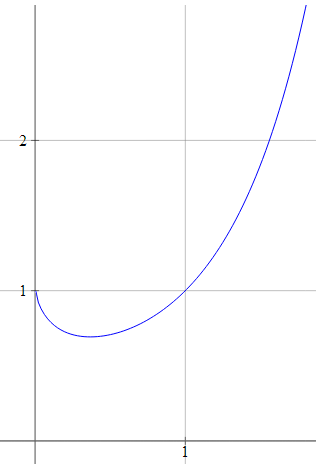32. Raoul says:

I might be getting too deep and meaningful, but here goes anyway.

I'm no mathematician, but I very much enjoy abstract concepts and philosophical thought.

Here is my postulation on whether 0x0 = 1.

If zero is representative of "oblivion" then we are talking about non-extistence. Non-existence cannot be multiplied by itself, because there is no itself.

If, however we are using zero as a placeholder in an equation, then it manages to EXIST as a concept. It therefore IS, and can therefore be multiplied by itself. It is, in that case, itself being one.

33. Murray says:

34. James Collins says:

I find this intriguing that after all these years there is a debate about getting 1 from nothing. Since any number multiplied by zero is zero . Zero to the zeroth power should also be zero. Since mathematics is logic based. Even the square root of negative one is "i" then you have I^4=1 .As far as anything to the 0th power being 1 , 0 itself should be the exception.

35. Jeremiah Johnson says:

There are a couple of ways to look at this, but we know conclusively that 0^0 is undefined. Consider for a moment what a 0 power is. This something to a zero dimension. Anything to a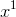is the first dimension, or a line, which we usually just write as a number and drop the "^1".

A zero dimension represented on a 2-dimensional space is a point.

When you take all values for a zero power, except 0, you will only see a point. But when you have a 0, the point is gone and nothing exists. So the value can be represented by either undefined(nothing) or 1.

There is another expression that is similar which is by taking the (n)th root of 0. All positive values for n = 0. Yet 0 and negative values yield undefined. However, the two are not equal. You can read more on the blog linked.

36. Theo Holmes says:

x=0^0
(The zero root of x)=0
x^(1/0)=0
x^(infinity)=0
what raised to the infinite power = 0?
Certainly 1 doesn't.
0*0*0*0*0... does.
0^0=0.
Algebra usually proves best in these kinds of things.

37. Jeremiah Johnson says:

@Theo Holmes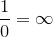.

This, however, is not true.

Consider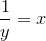This is the same as: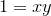But if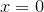then it becomes: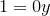Can any value for y make this true?

This is why mathematicians say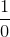is undefined.

38. Kae Verens says:

not a mathematician, but it occurs to me that a simple explanation is that x^y is "1 multiplied by x, y times", not "x multiplied by itself y times"

0^3 is 1*0*0*0 = 0
0^2 is 1*0*0 = 0
0^1 is 1*0 = 0
0^0 is 1 = 1

I'm wearing my flame-retardant t-shirt today, so fire away

39. Virat says:

Lim(x->0+) x^x is 1
But Lim(x->0-) x^x is undefined
So nothing can be said
(I really wonder how such math experts can make this mistake)

40. Serouj Ghazarian says:

Let's mix the complex values into this mess:
lim(x->0-)x^x=lim(x->0-)e^(xlnx)=e^((0-)×ln(0-))=e^((0-)×(ln(0+)+iπ)=e^(-(0+)×ln(0+))=e^(0+)=1+
Still 1.

41. ArnoldKarr says:

Another way to frame the problem is to start with the expression: x^a, which means x multiplied by itself a times. So x^2, for instance, means x*x or x used as a factor 2 times.
But that leads to a question of interpretation with a=1 and a=0 because it doesn't make clear sense to speak of multiplying a number by itself, where it is a factor 1 or 0 times. No multiplication seems to be taking place in either case.
However, if we restate the formula as x^a=1*1*x*... such that x appears as a factor a times, then 2^2=1*1*2*2=4; 2^1=1*1*2=2; 2^0=1*1=1, since 2 is a factor 0 times, but there is an actual multiplication taking place.
This way of stating it leads to the case of 0 as 0^2=1*1*0*0=0; 0^1=1*1*0=0; and 0^0=1*1=1, which is not the answer I like--I prefer to say 0^0 is undefined--but it doesn't clash with the common understanding of what exponents mean as a given number of factors of the same number in a multiplication sentence.

42. Yash says:

0^0 is 0 because suppose you multiply 5^2 * 0^0
5*0^(2+0) which is 0^2 which is 0.

### Comment Preview

HTML: You can use simple tags like <b>, <a href="...">, etc.

To enter math, you can can either:

1. Use simple calculator-like input in the following format (surround your math in backticks, or qq on tablet or phone):
a^2 = sqrt(b^2 + c^2)
(See more on ASCIIMath syntax); or
2. Use simple LaTeX in the following format. Surround your math with $$ and $$.
$$\int g dx = \sqrt{\frac{a}{b}}$$
(This is standard simple LaTeX.)

NOTE: You can mix both types of math entry in your comment.